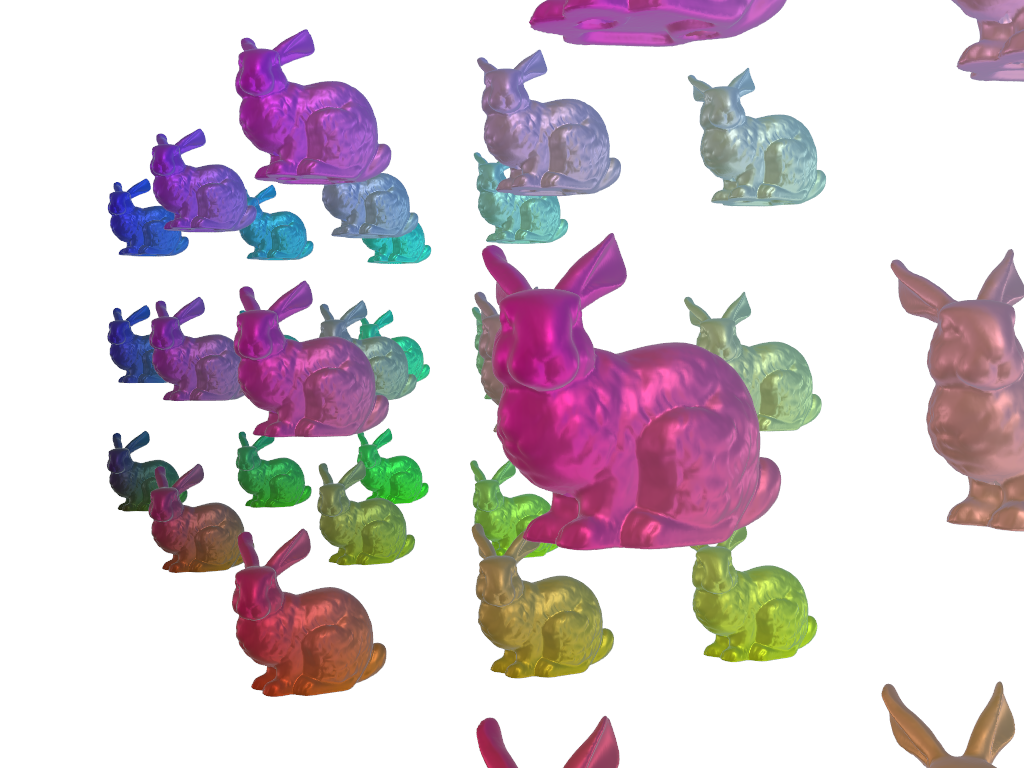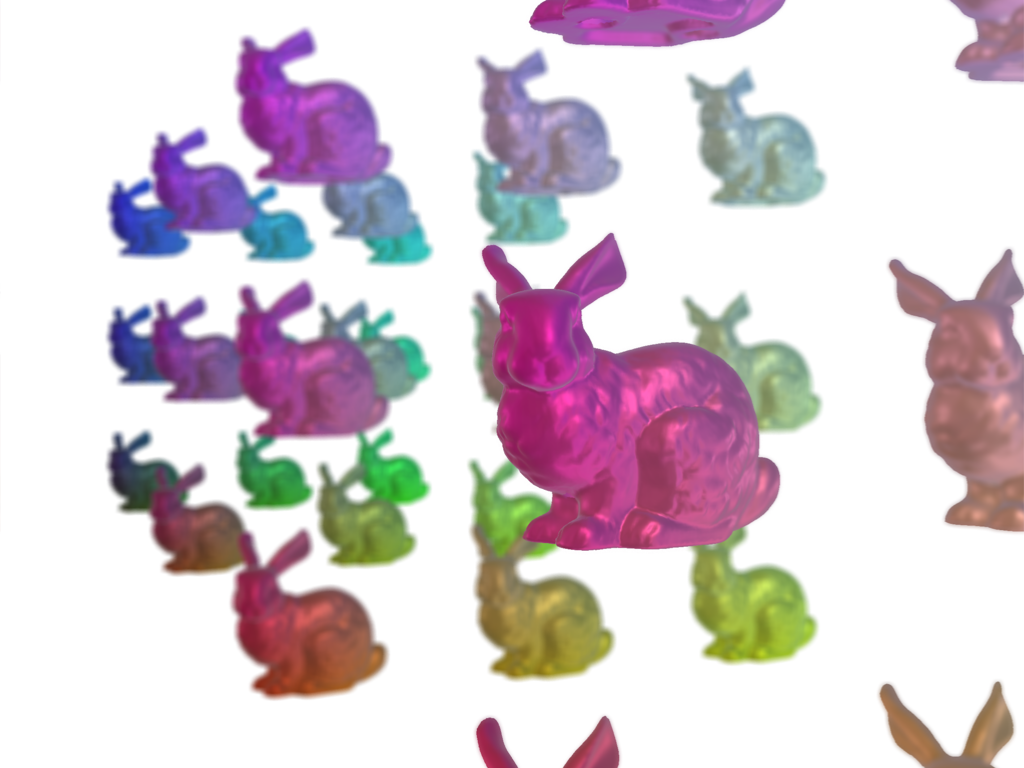# Depth of Field Plotting#

This example shows how you can use `enable_depth_of_field` to highlight part of your plot.

```import numpy as np

import pyvista as pv
from pyvista import examples
```

# Generate a bunch of bunnies#

Create many bunnies using the `glyph` filter.

```# download the stanford bunny and rotate it into a good position
mesh = mesh.rotate_x(90, inplace=False).rotate_z(90, inplace=False).scale(4, 4, 4)

# We use a uniform grid here simply to create equidistantly spaced points for
# our glyph filter
grid = pv.UniformGrid(dimensions=(4, 3, 3), spacing=(3, 1, 1))

bunnies = grid.glyph(geom=mesh, scale=False, orient=False)
bunnies
```
PolyDataInformation
N Cells2500236
N Points1294092
N Strips0
X Bounds-2.475e-01, 9.235e+00
Y Bounds-3.788e-01, 2.244e+00
Z Bounds1.319e-01, 2.749e+00
N Arrays0

# Show the plot without enabling depth of field#

```# convert points into rgba colors
colors = bunnies.points - bunnies.bounds[::2]
colors /= colors.max(axis=0)
colors *= 255
colors = colors.astype(np.uint8)

# obtained camera position with `cpos = pl.show(return_cpos)`
cpos = [(11.6159, -1.2803, 1.5338), (4.1354, 1.4796, 1.2711), (-0.0352, -0.0004, 1.0)]

# Since we're using physically based rendering (PBR), let's also download a
# skybox cubemap use it as an environment texture. For PBR to work well you
# should have a environment texture.

pl = pv.Plotter()
pl.background_color = 'w'
pl.camera_position = cpos
pl.set_environment_texture(cubemap)
pl.show()
```# Show the plot while enabling depth of field#

```pl = pv.Plotter()
pl.background_color = 'w'